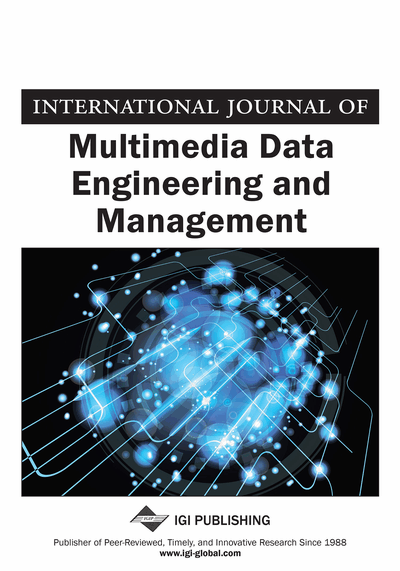# A Texture Preserving Image Interpolation Algorithm Based on Rational Function

Hongwei Du (Shandong University of Finance and Economics, Jinan, CN), Yunfeng Zhang (Shandong University of Finance and Economics, Jinan, CN), Fangxun Bao (Shandong University, Jinan, CN), Ping Wang (Shandong University of Finance and Economics, Jinan, CN) and Caiming Zhang (Shandong University, Jinan, CN)
DOI: 10.4018/IJMDEM.2018040103

## Abstract

In this article, a type of bivariate rational interpolation function is constructed for preserving image texture structure, which integrates polynomial functions with a rational function. On the basis of this model, an image interpolation algorithm for texture preserving is proposed. First, an isoline method is employed to detect the image texture, and then the image can be divided into texture regions and smooth regions adaptively. Second, the smooth region and the textured region are interpolated by the polynomial model and the rational model, respectively. Finally, in order to preserve image texture direction, an objective function based on the gradient is constructed, and the weight of the correlation point is calculated, and the pixel value of the interpolation point is determined by convolution. Experimental results show that the proposed algorithm achieves good competitive performance compared with the state-of-the-art interpolation algorithms, especially in preserving image details and edge structure.
Article Preview
Top

## Introduction

Image interpolation is the art of upscaling a low-resolution (LR) image to the high resolution (HR) version, which has become a very active area of research in image processing (Liu, Zhao, Zhou, Gao & Sun, 2014). It can be easily extended to diverse applications ranging from medical imaging, remote sensing, aviation, animation production and multimedia entertainment industries. Because of the relatively low computational complexity, traditional image interpolation methods such as bilinear, cubic spline and cubic convolution interpolations (Keys, 1981; Hou & Andrews, 1978; Jain, 1989) are widely used. But they often suffer from various forms of artifacts, such as blurring, ringing, jaggy edges and so on.

In general, the interpolation algorithm can be divided into discrete method and continuous method. The discrete method obtains the pixel value of the interpolated point by transforming the gray value of the known pixel. A new image interpolation method (Carrato & Tenze, 2000) which uses the priori edge model to optimize the parameters of the interpolation operator is proposed, and the high quality interpolation image is obtained by interpolating the edge information. New edge-directed interpolation (Li & Orchard, 2001) (NEDI) makes use of geometric duality property to estimate HR covariance by LR covariance, and interpolates the HR pixels using the estimated covariance. The method can effectively preserve the image edge structure. However, the interpolation window cannot be adjusted adaptively, which leads to distortion and deformation of the image texture details. Directional filtering and data fusion (Zhang & Wu, 2006) (DFDF) is a new edge-guided non-linear interpolation algorithm. For each pixel to be interpolated, its neighborhood is divided into two observation subsets, and then the missing pixels are estimated from the two orthogonal directions. The algorithm can preserve sharp edges of the image and reduce ring artifacts effectively. Soft-decision adaptive interpolation (Zhang & Wu, 2008) (SAI) adapts to varying scene structures by using a 2-D piecewise autoregressive model which interpolate missing pixels in groups rather than one. This method preserves the spatial coherence of the interpolated images, and achieves better effect in a wide range of scenarios. On the basis of SAI, robust soft-decision adaptive interpolation (Hung & Siu, 2012) (RSAI) improves the robustness of SAI by using weighted least squares estimation. Most of the adaptive interpolation based on discrete method cannot magnify at any multiple, preserve texture details effectively, prone to some artifacts (blur, ringing, zigzag, etc.). Further, combining with the machine learning method, Dong et al. developed an image interpolation method (Dong, Zhang, Lukac & Shi, 2013), wherein nonlocal autoregressive modeling (NARM) is embedded in the sparse representation model. The method achieves outstanding results compared to conventional edge-directed algorithms. However, it has higher time complexity.

Compared with the discrete method, the continuous method can construct a continuous surface with the image sampling data. The method can amplify any multiple. Because the natural image has nonlinear properties and the rational function is typical nonlinear model, the rational function interpolation algorithm has attracted attention of scholars (Lee, Pedersen & Mumford, 2003), and some research achievements have been made in recent years (Zhang, Zhang, Chi & Zhang, 2009; Yao, Zhang, Bao & Zhang, 2016; Yao, X., Zhang, Bao, Liu & Zhang, 2017; Zhang, Yao, Bao & Zhang, 2017). These algorithms are applied to the image enlargement and enhancement, and achieve significant results. Zhao et al. (Zhao, Hu & Tan, 2006) extended the 1-dimensional multi-node spline interpolation method to 2-dimensional, and then proposed a multi-node spline image interpolation algorithm. It is superior to the traditional cubic convolution interpolation algorithm. Liu et al. (Liu, Zhang, Guo & Zhang, 2014) proposed a mixed weighted rational function image interpolation model. The algorithm can maintain image structure information effectively. However, most of rational interpolation model iscontinuous, results are unsatisfying in preserving edge structure of image.

## Complete Article List

Search this Journal:
Reset
Open Access Articles: Forthcoming
Volume 11: 4 Issues (2020): 1 Released, 3 Forthcoming
Volume 10: 4 Issues (2019)
Volume 9: 4 Issues (2018)
Volume 8: 4 Issues (2017)
Volume 7: 4 Issues (2016)
Volume 6: 4 Issues (2015)
Volume 5: 4 Issues (2014)
Volume 4: 4 Issues (2013)
Volume 3: 4 Issues (2012)
Volume 2: 4 Issues (2011)
Volume 1: 4 Issues (2010)
View Complete Journal Contents Listing